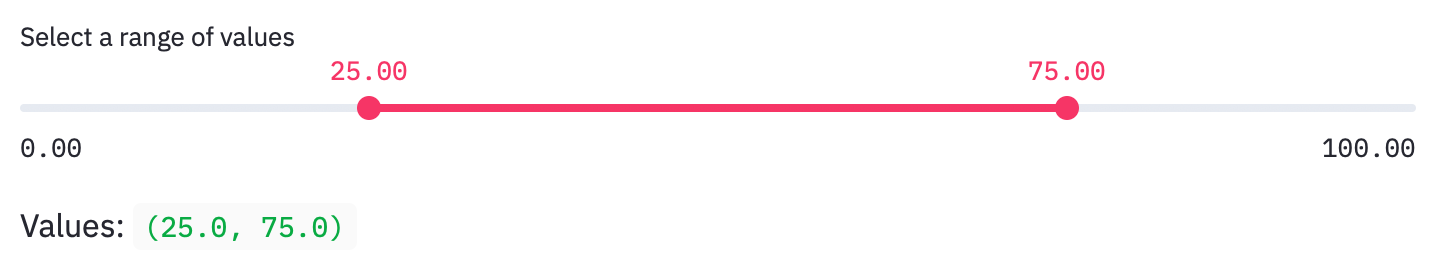# What is Streamlit

This article explains how to use Streamlit. Streamlit is a python framework that allows you to create front-end applications. Graphs created with Pandas DataFrame and drawing libraries such as plotly and altair can be embedded, and can be applied to data analysis depending on the device.

# environment

• macOS Catalina (ver.10.15.6)

# Installation

It can be installed in several ways.

1. Install with pip. Official HP Recommended

#### `Terminal`

``````
pip install streamlit
``````
1. Build a virtual environment with conda. Refer to the official forum

#### `Terminal`

``````
conda create -y -n streamlit python=3.7
conda activate streamlit
pip install streamlit
``````

# File execution

Execute the Python file as follows.

#### `Terminal`

``````
streamlit run filename.py
``````

# Streamlit features

The official HP API reference summarizes the features of streamlit. Among them, the functions that can be used for data analysis are summarized.

## Display title

#### `st_app.py`

``````
import streamlit as st
st.title('My app')
``````## View document

#### `st_app.py`

``````
import streamlit as st
st.write("Good morning")
``````## Displaying DataFrame

#### `st_app.py`

``````
import streamlit as st
import pandas as pd
st.table(pd.DataFrame({
'first column': [1, 2, 3, 4],
'second column': [10, 20, 30, 40]
}))
``````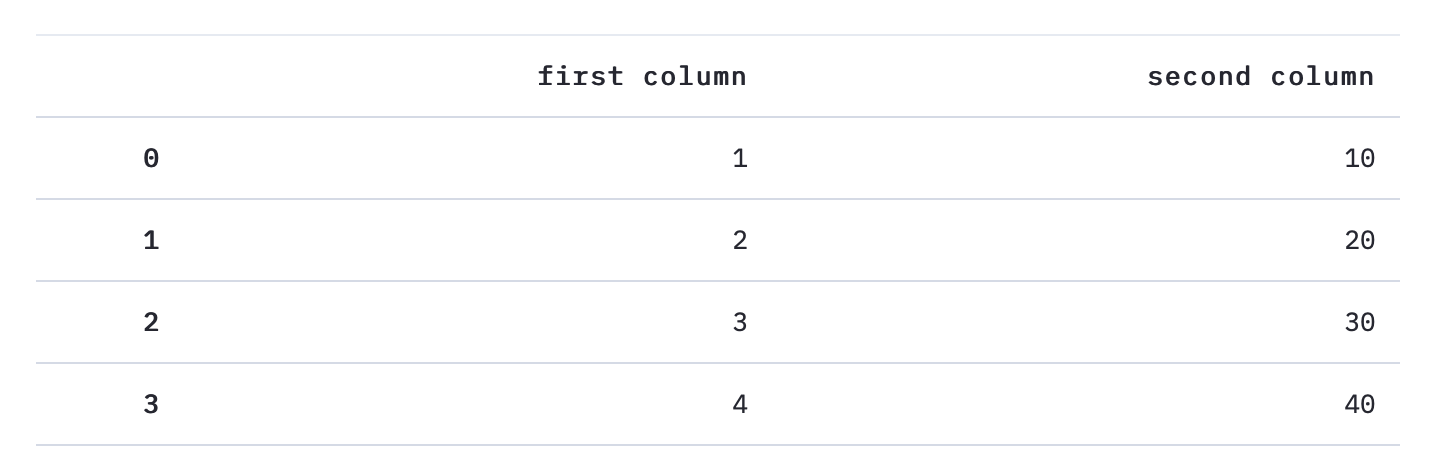## Markdown display

#### `st_app.py`

``````
import streamlit as st
st.markdown('# Markdown documents')
``````

## View Plotly

Plotly is one of the python drawing libraries that can create various types of graphs.

#### `st_app.py`

``````
import streamlit as st
import plotly.graph_objs as go

animals = ['giraffes', 'orangutans', 'monkeys']
populations = [20, 14, 23]

fig = go.Figure(data=[go.Bar(x=animals, y=populations)])

fig.update_layout(
xaxis = dict(
tickangle = 0,
title_text = "Animal",
title_font = {"size": 20},
title_standoff = 25),
yaxis = dict(
title_text = "Populations",
title_standoff = 25),
title ='Title')

st.plotly_chart(fig, use_container_width=True)

``````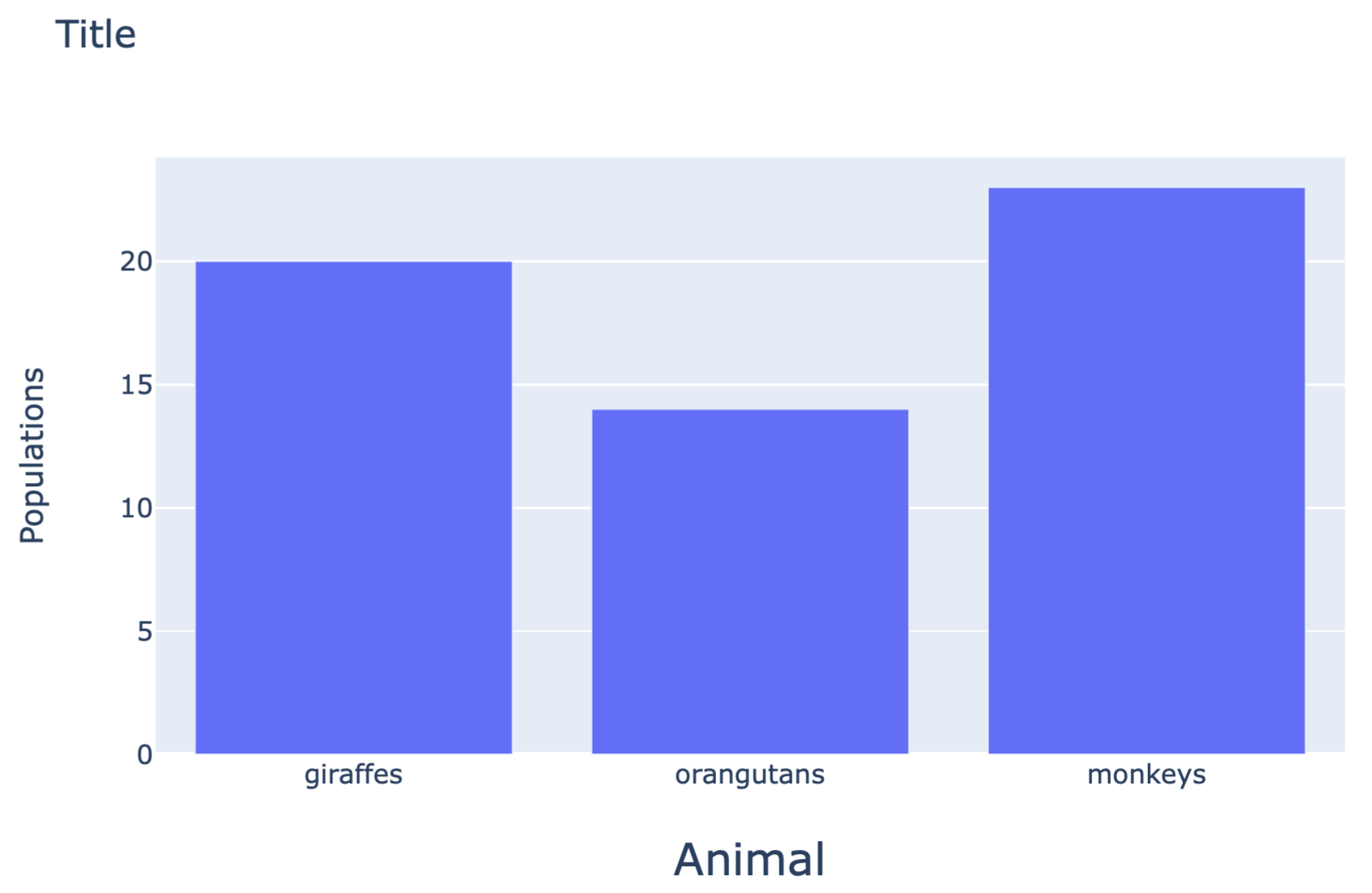## Display of Altair

Altair is one of the python drawing libraries that can create various types of graphs. It is characterized by inputting data with Pandas DataFrame. Reference

#### `st_app.py`

``````
import streamlit as st
import altair as alt
from vega_datasets import data

source = data.cars()

fig = alt.Chart(source).mark_circle(size=60).encode(
x='Horsepower',
y='Miles_per_Gallon',
color='Origin',
tooltip=['Name', 'Origin', 'Horsepower', 'Miles_per_Gallon']
).properties(
width=500,
height=500
).interactive()

st.write(fig)
``````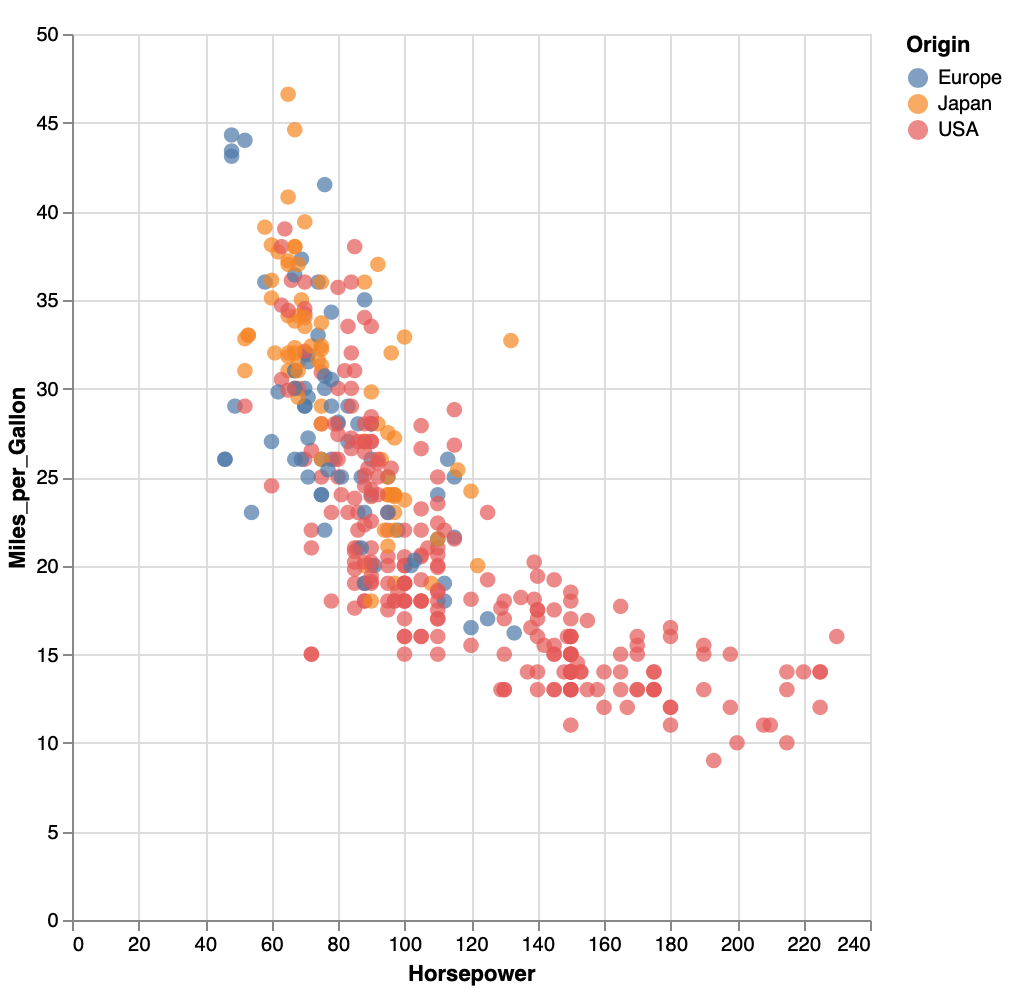## Button display

Altair can display a button that returns a bool type.

#### `st_app.py`

``````
import streamlit as st
answer = st.button('Say hello')

if answer == True:
st.write('Why hello there')
else:
st.write('Goodbye')
``````

[Before pressing][After pressing]## Check button display

It is also possible to display a check button that returns a bool type.

#### `st_app.py`

``````
import streamlit as st
answer = st.button('Say hello')

if answer == True:
st.write('Why hello there')
else:
st.write('Goodbye')
``````

【no check】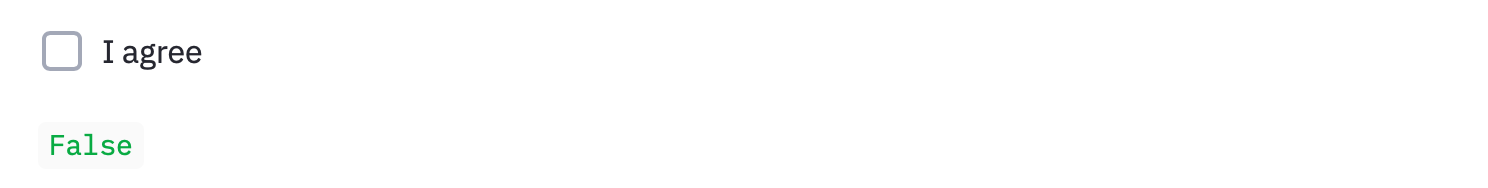[Checked]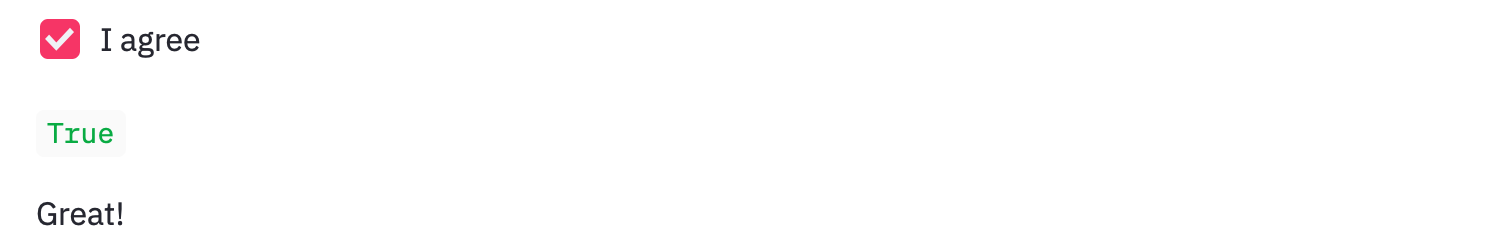## Radio button display

It is also possible to select an element with a radio button.

#### `st_app.py`

``````
import streamlit as st
"What's your favorite movie genre",
('Comedy', 'Drama', 'Documentary'))

if genre == 'Comedy':
st.write('You selected comedy.')
else:
st.write("You didn't select comedy.")
``````## Dropdown display

If you want to select only one from the dropdown:

#### `st_app.py`

``````
import streamlit as st
agree = st.checkbox('I agree')

if agree == True :
st.write('Great!')
``````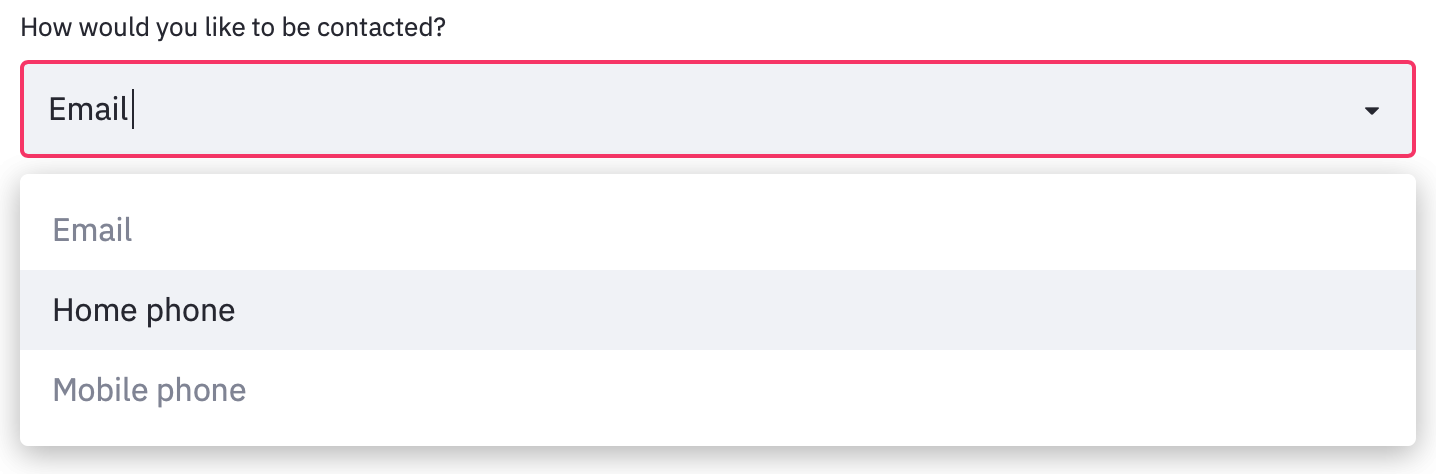To select two or more from the dropdown at the same time:

#### `st_app.py`

``````
import streamlit as st
options = st.multiselect(
'What are your favorite colors',
['Green', 'Yellow', 'Red', 'Blue'],
['Yellow', 'Red'])

st.table(options)
``````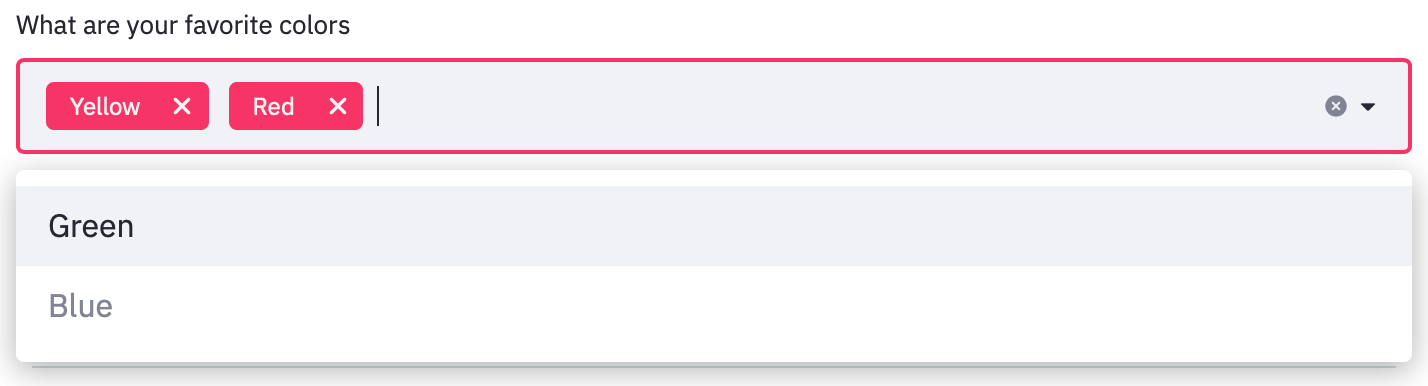## Slider display

To select one value: In the following example, a slider that moves with a minimum value of 0, a maximum value of 130, an interval of 1, and an initial value of 25 is displayed.

#### `st_app.py`

``````
import streamlit as st
age = st.slider('How old are you?',  min_value=0, max_value=130, step=1, value=25)
st.write("I'm ", age, 'years old')
``````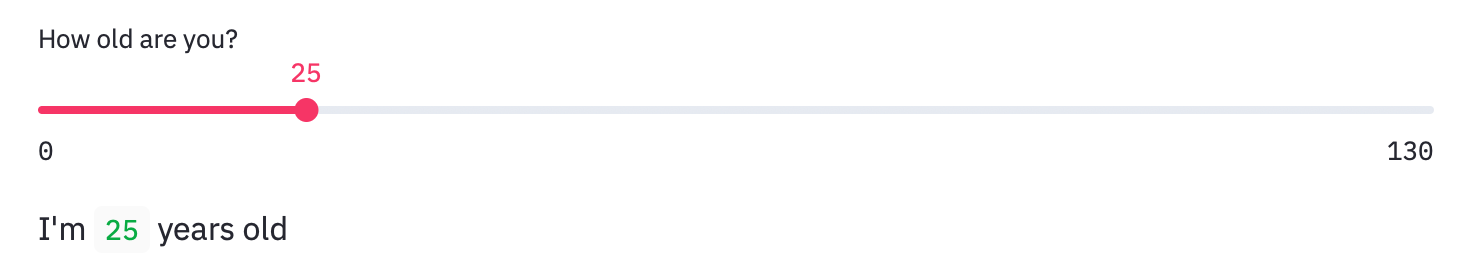To select two values: In the following example, a slider that moves with a minimum value of 0.0, a maximum value of 100.0, and an initial value of `(25.0, 75.0)` is displayed.

#### `st_app.py`

``````
import streamlit as st
values = st.slider(
'Select a range of values',
0.0, 100.0, (25.0, 75.0))
st.write('Values:', values)
``````